# 15  Serial Data

For more information see RMS Chapter 7

## 15.1 Introduction

A

Serial data, also called longitudinal data, repeated measures, or panel data, present a special challenge in that the response variable is multivariate, and that the responses measured at different times (or other conditions such as doses) but on the same subject are correlated with each other. One expects correlations between two measurements measured closer together will exceed correlations for two distant measurements on the same subject. Analyzing the repeated measurements just as though they were independent measurements falsely inflates the sample size and results in a failure to preserve type I error and confidence interval coverage. For example, having three measurements on 10 subjects results in 30 measurements, but depending on the correlations among the three measurements within subject, the effective sample size might be 16, for example. In other words, the statistical information for collecting 3 measurements on each of 10 subjects might provide the same statistical information and power as having one measurement on each of 16 subjects. The statistical information would however be less than that from 30 subjects measured once, if the intra-subject correlation exceeds zero.

The most common problems in analyzing serial data are

B
1. treating repeated measurements per subject as if they were from separate subjects
2. using two-way ANOVA as if different measurement times corresponded to different groups of subjects
3. using repeated measures ANOVA which assumes that the correlation between any two points within subject is the same regardless of how far apart the measures were timed
4. analyzing more than 3 time points as if time is a categorical rather than a continuous variable
• multiplicity problem
• analyses at different times may be inconsistent since times are not connected
• loss of power by not jointly analyzing the serial data

## 15.2 Analysis Options

There are several overall approaches to the analysis of serial measurements. Some of the older approaches such as multiple $$t$$-tests and repeated measures ANOVA are now considered obsolete because of the availability of better methods that are more flexible and robust1. Separate $$t$$-tests at each time point do not make good use of available information, use an inefficient estimate of $$\sigma^2$$, do not interpolate time points, and have multiple comparison problems. Since a multiplicity adjustment for multiple correlated $$t$$-tests is not model-based, the resulting confidence intervals and $$P$$-values are conservative. To preserve type I error, one always must sacrifice type II error, but in this case the sacrifice is too severe. In addition, investigators are frequently confused by the $$t$$-test being “significant” at one time point and not at another, and make the unwarranted claim that the treatment is effective only at the first time. Besides not recognizing the absence of evidence is not evidence for absence problem, the investigator can be mislead by increased variability in response at the second time point driving the $$t$$ ratio towards zero. This variability may not even be real but may reflect instability in estimating $$\sigma$$ separately at each time point.

C
• 1 Repeated measures ANOVA makes stringent assumptions and requires complex adjustments for within-subject correlation.

• Especially when there are more than three unique measurement times, it is advisable to model time as a continuous variable. When estimation of the time-response profile is of central importance, that may be all that’s needed. When comparing time-response profiles (e.g., comparing two treatments) one needs to carefully consider the characteristics of the profiles that should be tested, i.e., where the type I and II errors should be directed, for example

D
• difference in slope
• difference in area under the curve
• difference in mean response at the last planned measurement time
• difference in mean curves at any time, i.e., whether the curves have different heights or shapes anywhere

The first 3 are 1 d.f. tests, and the last is a 2 d.f. test if linearity is assumed, and $$> 2$$ d.f. if fitting a polynomial or spline function.

### 15.2.1 Joint Multivariate Models

E

This is the formal fully-specified statistical model approach whereby a likelihood function is formed and maximized. This handles highly imbalanced data, e.g., one subject having one measurement and another having 100. It is also the most robust approach to non-random subject dropouts. These two advantages come from the fact that full likelihood models “know” exactly how observations from the same subject are connected to each other.

Examples of full likelihood-based models include generalized least squares (GLS), mixed effects models, and Bayesian hierarchical models. GLS only handles continuous $$Y$$ and assumes multivariate normality. It does not allow different subjects to have different slopes. But it is easy to specify and interpret, to have its assumptions checked, and it runs faster. Mixed effects models can handle multiple hierarchical levels (e.g., state/hospital/patient) and random slopes whereby subjects can have different trajectories. Mixed effects models can be generalized to binary and other endpoints but lose their full likelihood status somewhat when these extensions are used, unless a Bayesian approach is used.

• Mixed effects model have random effects (random intercepts and possibly also random slopes or random shapes) for subjects
• These allow estimation of the trajectory for an individual subject
• When the interest is instead on group-level effects (e.g., average difference between treatments, over subjects), GLS squares models may be more appropriate
• The generalization of GLS to non-normally distributed $$Y$$ is marginalized models (marginalized over subject-level effects)

GLS, formerly called growth curve models, is the oldest approach and has excellent performance when $$Y$$ is conditionally normally distributed.

### 15.2.2 GEE

Generalized estimating equations is usually based on a working independence model whereby ordinary univariate regressions are fitted on a combined dataset as if all observations are uncorrelated, and then an after-the-fit correction for intra-cluster correlation is done using the cluster sandwich covariance estimator or the cluster bootstrap. GEE is very non-robust to non-random subject dropout; it assumes missing response values are missing completely at random. It may also require large sample sizes for $$P$$-values and confidence intervals to be accurate. An advantage of GEE is that it extends easily to every type of response variable, including binary, ordinal, polytomous, and time-to-event responses.

F

### 15.2.3 Summary Measures

A simple and frequently effective approach is to summarize the serial measures from each subject using one or two measures, then to analyze these measures using traditional statistical methods that capitalize on the summary measures being independent for different subjects. This has been called

GH
1. Two-stage derived variable analysis
2. Response feature analysis
3. Longitudinal analysis through summary measures

An excellent overview may be found in Matthews et al. (1990), Dupont (2008) (Chapter 11), and Senn et al. (2000).

Frequently chosen summary measures include the area under the time-response curve, slope, intercept, and consideration of multiple features simultaneously, e.g., intercept, coefficient of time, coefficient of time squared when fitting each subject’s data with a quadratic equation. This allows detailed analyses of curve shapes.

## 15.3 Case Study

### 15.3.1 Data and Summary Measures

Consider the isoproterenol dose-response analysis of the original data from Lang2. Twenty two normotensive men were studied, 9 of them black and 13 white. Blood flow was measured before the drug was given, and at escalating doses of isoproterenol. Most subjects had 7 measurements, and these are not independent. Note: Categorization of race is arbitrary and represents a gross oversimplification of genetic differences in physiology.

I
• 2 CC Lang etal NEJM 333:155-60, 1995

• Code
require(Hmisc)
require(data.table)   # elegant handling of aggregation
require(ggplot2)
d <- csv.get('https://hbiostat.org/data/repo/11.2.Long.Isoproterenol.csv')
d <- upData(d, keep=c('id', 'dose', 'race', 'fbf'),
race  =factor(race, 1:2, c('white', 'black')),
labels=c(fbf='Forearm Blood Flow'),
units=c(fbf='ml/min/dl'))
Input object size:   13168 bytes;    8 variables     154 observations
Modified variable   race
Kept variables  id,dose,race,fbf
New object size:    6496 bytes; 4 variables 154 observations
Code
setDT(d, key=c('id', 'race'))

J
Code
# Fit subject-by-subject spline fits and either return the coefficients,
# the estimated area under the curve from [0,400], or evaluate each
# subject's fitted curve over a regular grid of 150 doses
# Area under curve is divided by 400 to get a mean function
require(rms)
options(prType='html')
g <- function(x, y, what=c('curve', 'coef', 'area')) {
what <- match.arg(what)   # 'curve' is default
knots <- c(20, 60, 150)
f <- ols(y ~ rcs(x, knots))
xs <- seq(0, 400, length=150)
switch(what,
coef = {k <- coef(f)
list(b0 = k, b1=k, b2=k)},
curve= {x <- seq(0, 400, length=150)
list(dose=xs, fbf=predict(f, data.frame(x=xs)))},
area = {antiDeriv = rcsplineFunction(knots, coef(f),
type='integral')
list(dose  = 400, fbf=y[x == 400],
area  = antiDeriv(400) / 400,
tarea = areat(x, y) / 400)} )
}
# Function to use trapezoidal rule to compute area under the curve
areat <- function(x, y) {
i <- ! is.na(x + y)
x <- x[i]; y <- y[i]
i <- order(x)
x <- x[i]; y <- y[i]
if(! any(x == 400)) NA else
sum(diff(x) * (y[-1] + y[-length(y)]))/2
}

w <- d[, j=g(dose, fbf), by = list(id, race)]   # uses data.table package
a <- d[, j=g(dose, fbf, what='area'), by = list(id, race)]

ggplot(d, aes(x=dose, y=fbf, color=factor(id))) +
geom_line() + geom_line(data=w, alpha=0.25) +
geom_text(aes(label = round(area,1)), data=a, size=2.5,
position=position_dodge(width=50)) +
xlab('Dose') + ylab(label(d$fbf, units=TRUE, plot=TRUE)) + facet_grid(~ race) + guides(color=FALSE)Figure 15.1: Spaghetti plots for isoproterenol data showing raw data stratified by race. Next to each curve is the area under the curve divided by 400 to estimate the mean response function. The area is computed analytically from a restricted cubic spline function fitted separately to each subject’s dose-response curve. Shadowing the raw data are faint lines depicting spline fits for each subject K Code ggplot(a, aes(x=tarea, y=area, color=race)) + geom_point() + geom_abline(col=gray(.8)) + xlab('Area by Trapezoidal Rule / 400') + ylab('Area by Spline Fit / 400')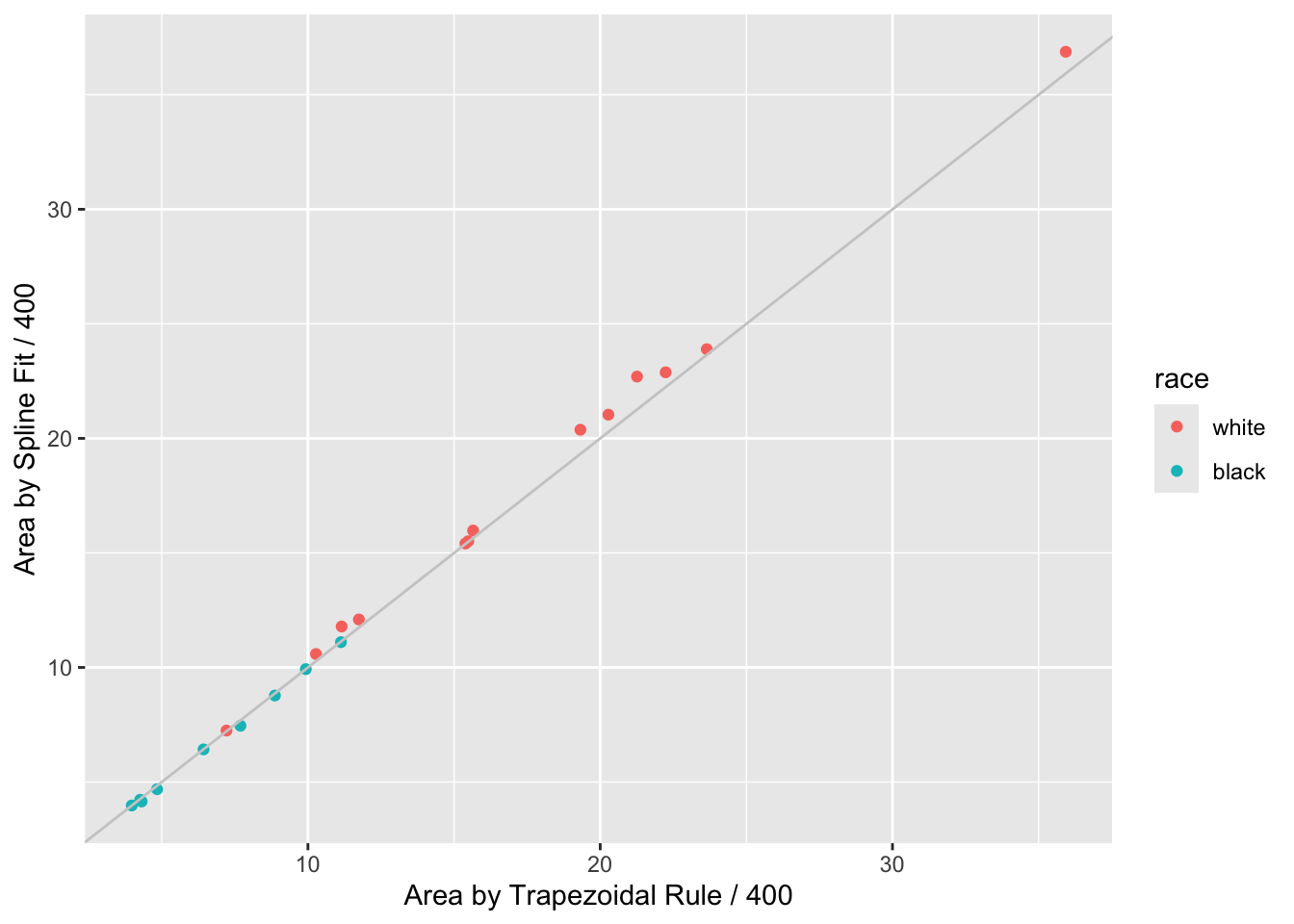Figure 15.2: AUC by curve fitting and by trapezoidal rule When a subject’s dose (or time) span includes the minimum and maximum values over all subjects, one can use the trapezoidal rule to estimate the area under the response curve empirically. When interior points are missing, linear interpolation is used. The spline fits use nonlinear interpolation, which is slightly better, as is the spline function’s assumption of continuity in the slope. @ref(fig-serial-auctwo) compares the area under the curve (divided by 400 to estimate the mean response) estimated using the the two methods. Agreement is excellent. In this example, the principle advantage of the spline approach is that slope and shape parameters are estimated in the process, and these parameters may be tested separately for association with group (here, race). For example, one may test whether slopes differ across groups, and whether the means, curvatures, or inflection points differ. One could also compare AUC from a sub-interval of $$X$$. L Code ggplot(a, aes(x=race, y=area)) + geom_boxplot(alpha=.5, width=.25) + geom_point() + coord_flip() + ylab(expression(paste('Mean Forearm Blood Flow, ', scriptstyle(ml/min/dl))))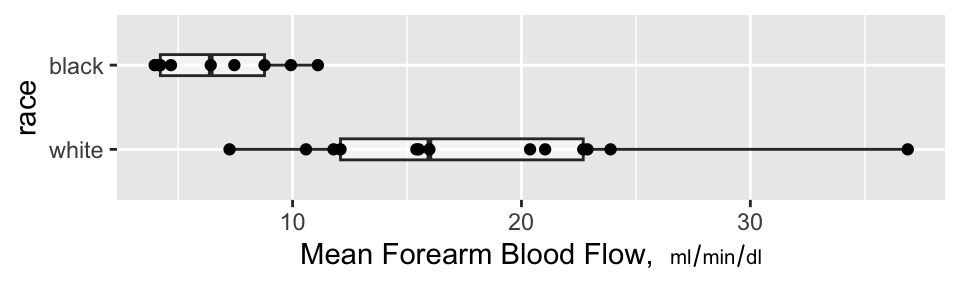Figure 15.3: Mean blood flow computed from the areas under the spline curves, stratified by race, along with box plots ### 15.3.2 Nonparametric Test of Race Differences in AUC A minimal-assumption approach to testing for differences in isoproterenol dose-response between races is to apply the Wilcoxon test to the normalized AUCs (mean response functions). M Code wilcox.test(area ~ race, data=a, conf.int=TRUE)  Wilcoxon rank sum exact test data: area by race W = 112, p-value = 7.639e-05 alternative hypothesis: true location shift is not equal to 0 95 percent confidence interval: 5.670113 16.348121 sample estimates: difference in location 11.25836  There is strong evidence that the mean response is greater for whites. ### 15.3.3 Nonparametric Test of General Curve Differences O’Brien (1988) proposed a method for using logistic regression to turn the Hotelling $$T^2$$ test on its side. The Hotelling test is the multivariate analog of the two-sample $$t$$-test, and can be used to test simultaneously such things as whether a treatment modifies either systolic or diastolic blood pressure. O’Brien’s idea was to test whether systolic or diastolic blood pressure (or both) can predict which treatment was given. Here we use the idea to test for race differences in the shape of the dose-response curves. We do this by predicting race from a 3-predictor model—one containing the intercept, the next the coefficient of the linear dose effect and the third the coefficient of the nonlinear restricted cubic spline term (differences in cubes). These coefficients were estimated using ordinary least squares in separately predicting each subject’s relationship between dose and forearm blood flow. N Code h <- d[, j=g(dose, fbf, what='coef'), by = list(id, race)] h  id race b0 b1 b2 1: 1 white -0.1264763 0.32310327 -0.34253352 2: 2 white 2.1147707 0.22798336 -0.21959542 3: 3 white 2.3378721 0.06927717 -0.03625384 4: 4 white 1.4822502 0.19837740 -0.20727584 5: 5 white 2.5366751 0.15399740 -0.14756999 6: 6 white 3.2117187 0.19910942 -0.18650561 7: 7 white 1.4264366 0.05261565 -0.03871545 8: 8 white 3.0999999 0.21833887 -0.19813457 9: 9 white 5.1507764 0.45026617 -0.48013920 10: 10 white 4.4778127 0.23853904 -0.23289815 11: 11 white 1.9052885 0.13548226 -0.13917910 12: 12 white 2.1828176 0.07558431 -0.05524955 13: 13 white 2.9318982 0.12776900 -0.10679867 14: 14 black 2.3336099 0.02679742 -0.02856275 15: 15 black 1.8356227 0.07652884 -0.07972036 16: 16 black 2.5342537 0.02290717 -0.02585081 17: 17 black 2.0254606 0.06002835 -0.06261969 18: 18 black 3.3279080 0.07620477 -0.08062536 19: 19 black 1.9308650 0.03844018 -0.04060065 20: 20 black 1.7263259 0.12358392 -0.13595538 21: 21 black 1.3215502 0.03528716 -0.03480467 22: 22 black 2.0828281 0.03143768 0.02251155 id race b0 b1 b2 Code f <- lrm(race ~ b0 + b1 + b2, data=h, x=TRUE, y=TRUE) f Logistic Regression Model  lrm(formula = race ~ b0 + b1 + b2, data = h, x = TRUE, y = TRUE)  Model Likelihood Ratio Test Discrimination Indexes Rank Discrim. Indexes Obs 22 LR χ2 19.73 R2 0.798 C 0.957 white 13 d.f. 3 R23,22 0.533 Dxy 0.915 black 9 Pr(>χ2) 0.0002 R23,16 0.650 γ 0.915 max |∂log L/∂β| 8×10-7 Brier 0.080 τa 0.463 β S.E. Wald Z Pr(>|Z|) Intercept 3.6618 4.1003 0.89 0.3718 b0 1.3875 2.5266 0.55 0.5829 b1 -165.3481 92.7864 -1.78 0.0747 b2 -101.1667 63.1549 -1.60 0.1092 The likelihood ratio $$\chi^{2}_{3}=19.73$$ has $$P=0.0002$$ indicating strong evidence that the races have different averages, slopes, or shapes of the dose-response curves. The $$c$$-index of 0.957 indicates nearly perfect ability to separate the races on the basis of three curve characteristics (although the sample size is small). We can use the bootstrap to get an overfitting-corrected index. O Code set.seed(2) v <- validate(f, B=1000) Divergence or singularity in 271 samples Code html(v) Index Original Sample Training Sample Test Sample Optimism Corrected Index $$n$$ $$D_{xy}$$ 0.9145 0.9589 0.8423 0.1166 0.798 729 $$R^{2}$$ 0.7985 0.9096 0.6617 0.2479 0.5506 729 Intercept 0 0 0.115 -0.115 0.115 729 Slope 1 1 0.4259 0.5741 0.4259 729 $$E_{\max}$$ 0 0 0.2056 0.2056 0.2056 729 $$D$$ 0.8513 1.0728 0.6434 0.4294 0.4219 729 $$U$$ -0.0909 -0.0909 2.608 -2.6989 2.608 729 $$Q$$ 0.9422 1.1637 -1.9646 3.1283 -2.1861 729 $$B$$ 0.0803 0.031 0.0773 -0.0463 0.1266 729 $$g$$ 6.7833 23.8206 4.4145 19.4061 -12.6228 729 $$g_{p}$$ 0.4712 0.4629 0.4255 0.0373 0.4339 729 The overfitting-corrected $$c$$-index is $$c = \frac{D_{xy} + 1}{2}$$ = 0.9. ### 15.3.4 Model-Based Analysis: Generalized Least Squares Generalized least squares (GLS) is the first generalization of ordinary least squares (multiple linear regression). It is described in detail in Regression Modeling Strategies Chapter 7 where a comprehensive case study is presented. The assumptions of GLS are PQ • All the usual assumptions about the right-hand-side of the model related to transformations of $$X$$ and interactions • Residuals have a normal distribution • Residuals have constant variance vs. $$\hat{Y}$$ or any $$X$$ (but the G in GLS also refers to allowing variances to change across $$X$$) • The multivariate responses have a multivariate normal distribution conditional on $$X$$ • The correlation structure of the conditional multivariate distribution is correctly specified With fully specified serial data models such as GLS, the fixed effects of time or dose are modeled just as any other predictor, with the only difference being that it is the norm to interact the time or dose effect with treatment or whatever $$X$$ effect is of interest. This allows testing hypotheses such as R • Does the treatment effect change over time? (time $$\times$$ treatment interaction) • Is there a time at which there is a treatment effect? (time $$\times$$ treatment interaction + treatment main effect combined into a chunk test) • Does the treatment have an effect at time $$t$$? (difference of treatments fixing time at $$t$$, not assuming difference is constant across different $$t$$) In the majority of longitudinal clinical trials, the last hypothesis is the most important, taking $$t$$ as the end of treatment point. This is because one is often interested in where patients ended up, not just whether the treatment provided temporary relief. S Now consider the isoproterenol dataset and fit a GLS model allowing for the same nonlinear spline effect of dose as was used above, and allowing the shapes of curves to be arbitrarily different by race. We impose a continuous time AR1 correlation structure on within-subject responses. This is the most commonly used correlation structure; it assumes that the correlation between two points is exponentially declining as the difference between two times or doses increases. We fit the GLS model and examine the equal variance assumption. T Code require(nlme) dd <- datadist(d); options(datadist='dd') a <- Gls(fbf ~ race * rcs(dose, c(20,60,150)), data=d, correlation=corCAR1(form = ~ dose | id)) plot(fitted(a), resid(a))Figure 15.4: Residual plot for generalized least squares fit on untransformed fbf The variance of the residuals is clearly increasing with increasing dose. Try log transforming both fbf and dose. The log transformation requires a very arbitrary adjustment to dose to handle zeros. Code a <- Gls(log(fbf) ~ race * rcs(log(dose + 1), log(c(20,60,150)+1)), data=d, correlation=corCAR1(form = ~ dose | id)) anova(a)  χ2 d.f. P Wald Statistics for log(fbf) race (Factor+Higher Order Factors) 99.54 3 <0.0001 All Interactions 19.30 2 <0.0001 dose (Factor+Higher Order Factors) 312.10 4 <0.0001 All Interactions 19.30 2 <0.0001 Nonlinear (Factor+Higher Order Factors) 2.01 2 0.3667 race × dose (Factor+Higher Order Factors) 19.30 2 <0.0001 Nonlinear 0.07 1 0.7969 Nonlinear Interaction : f(A,B) vs. AB 0.07 1 0.7969 TOTAL NONLINEAR 2.01 2 0.3667 TOTAL NONLINEAR + INTERACTION 21.16 3 <0.0001 TOTAL 391.48 5 <0.0001 There is little evidence for a nonlinear dose effect on the log scale, implying that the underlying model is exponential on the original $$X$$ and $$Y$$ scales. This is consistent with Dupont (2008). Re-fit the model as linear in the logs. Before taking this as the final model, also fit the same model but using a correlation pattern based on time rather than dose. Assume equal time spacing during dose escalation. U Code a <- Gls(log(fbf) ~ race * log(dose + 1), data=d, correlation=corCAR1(form = ~ dose | id)) d$time <- match(d\$dose, c(0, 10, 20, 60, 150, 300, 400)) - 1
b <- Gls(log(fbf) ~ race * log(dose + 1), data=d,
correlation=corCAR1(form = ~ time | id))
AIC(a);AIC(b)

 231.3731  161.3765

Code
b

Generalized Least Squares Fit by REML

 Gls(model = log(fbf) ~ race * log(dose + 1), data = d, correlation = corCAR1(form = ~time |
id))

Obs 150 Log-restricted-likelihood -74.69
Clusters 22 Model d.f. 3
g 0.755 σ 0.5023
d.f. 146
β S.E. t Pr(>|t|)
Intercept   0.9851  0.1376 7.16 <0.0001
race=black  -0.2182  0.2151 -1.01 0.3120
dose   0.3251  0.0286 11.38 <0.0001
race=black × dose  -0.1421  0.0446 -3.19 0.0018
 Correlation Structure: Continuous AR(1)
Formula: ~time | id
Parameter estimate(s):
Phi
0.6886846

Code
anova(b)
 χ2 d.f. P Wald Statistics for log(fbf) race (Factor+Higher Order Factors) 32.45 2 <0.0001 All Interactions 10.16 1 0.0014 dose (Factor+Higher Order Factors) 158.11 2 <0.0001 All Interactions 10.16 1 0.0014 race × dose (Factor+Higher Order Factors) 10.16 1 0.0014 TOTAL 180.17 3 <0.0001

Lower AIC is better, so it is clear that time-based correlation structure is far superior to dose-based. We will used the second model for the remainder of the analysis. But first we check some of the model assumptions.

V
Code
w <- data.frame(residual=resid(b), fitted=fitted(b))
p1 <- ggplot(w, aes(x=fitted, y=residual)) + geom_point()
p2 <- ggplot(w, aes(sample=residual)) + stat_qq()
gridExtra::grid.arrange(p1, p2, ncol=2)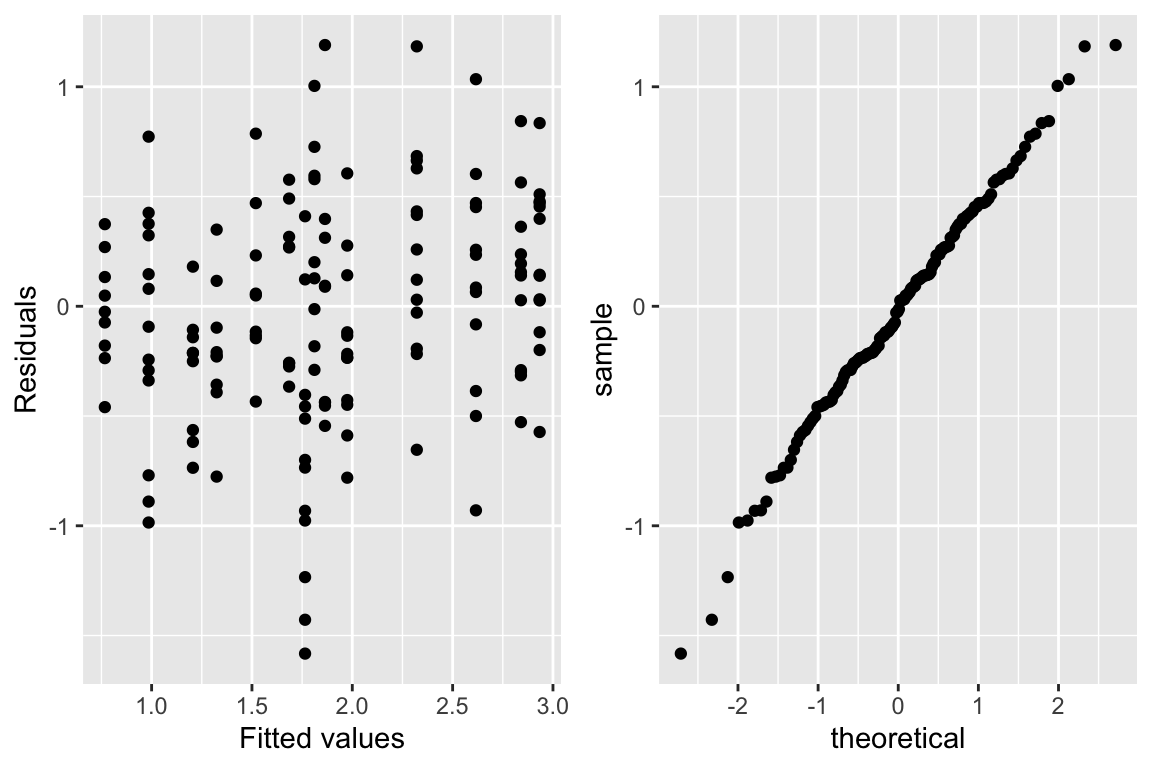Figure 15.5: Checking assumptions of the GLS model that is linear after logging dose and blood flow. The graph on the right is a QQ-plot to check normality of the residuals from the model, where linearity implies normality.

The test for dose $$\times$$ race interaction in the above ANOVA summary of Wald statistics shows strong evidence for difference in curve characteristics across races. This test agrees in magnitude with the less parametric approach using the logistic model above. But the logistic model also tests for an overall shift in distributions due to race, and the more efficient test for the combined race main effect and dose interaction effect from GLS is more significant with a Wald $$\chi^{2}_{2} = 32.45$$3. The estimate of the correlation between two log blood flows measured on the same subject one time unit apart is 0.69.

W
• 3 The $$\chi^2$$ for race with the dose-based correlation structure was a whopping 100 indicating that lack of fit of the correlation structure can have a significant effect on the rest of the GLS model.

• The equal variance and normality assumptions appear to be well met as judged by @ref(fig-serial-glsc).

Now estimate the dose-response curves by race, with pointwise confidence intervals and simultaneous intervals that allow one to make statements about the entire curves4. Anti-log the predicted values to get predictions on the original blood flow scale. Anti-logging predictions from a model that assumes a normal distribution on the logged values results in estimates of the median response.

• 4 Since the model is linear in log dose there are two parameters involving dose—the dose main effect and the race $$\times$$ dose interaction effect. The simultaneous inference adjustment only needs to reflect simultaneity in two dimensions.

• X
Code
dos <- seq(0, 400, length=150)
p <- Predict(b, dose=dos, race, fun=exp)
s <- Predict(b, dose=dos, race, fun=exp, conf.type='simultaneous')
ps <- rbind(Pointwise=p, Simultaneous=s)
ggplot(ps, ylab=expression(paste('Median Forearm Blood Flow,  ',
scriptstyle(ml/min/dl))))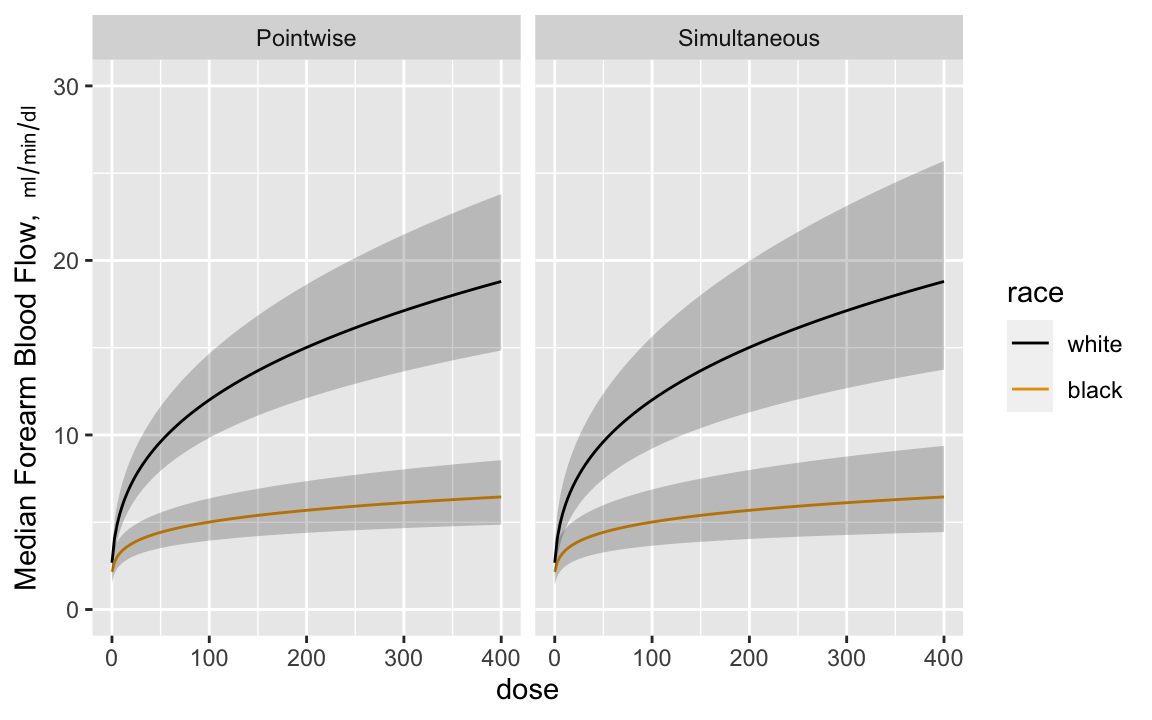Figure 15.6: Pointwise and simultaneous confidence bands for median dose-response curves by race

Finally we estimate the white:black fold change (ratio of medians) as a function of dose with simultaneous confidence bands.

Y
Code
k <- contrast(b, list(dose=dos, race='white'),
list(dose=dos, race='black'), conf.type='simultaneous')
k <- as.data.frame(k[c('dose', 'Contrast', 'Lower', 'Upper')])
ggplot(k, aes(x=dose, y=exp(Contrast))) + geom_line() +
geom_ribbon(aes(ymin=exp(Lower), ymax=exp(Upper)), alpha=0.2, linetype=0,
show_guide=FALSE) +
geom_hline(yintercept=1, col='red', size=.2) +
ylab('White:Black Ratio of Median FBF')Figure 15.7: White:black fold change for median response as a function of dose, with simultaneous confidence band

By comparing the simultaneous confidence binds to the red horizontal line, one can draw the inference that the dose-response for blacks is everywhere different than that for whites when the dose exceeds zero.

### 15.3.5 Longitudinal Bayesian Mixed Effects Semiparametric Model

• Proportional odds model with random effects
• Robust with regard to distribution
• Compound symmetry may not be realistic
• Model estimates logits of exceedance probabilities
• Convert these to means on the original scale
Code
require(rmsb)
options(mc.cores = parallel::detectCores() - 1)
b <- blrm(fbf ~ race * rcs(dose, 5) + cluster(id), data=d)
b

Bayesian Proportional Odds Ordinal Logistic Model

Dirichlet Priors With Concentration Parameter 0.023 for Intercepts

 blrm(formula = fbf ~ race * rcs(dose, 5) + cluster(id), data = d)


Frequencies of Missing Values Due to Each Variable

         fbf        race        dose cluster(id)
4           0           0           0

Mixed Calibration/
Discrimination Indexes
Discrimination
Indexes
Rank Discrim.
Indexes
Obs 150 LOO log L -769.56±17.76 g 6.615 [5.588, 7.644] C 0.865 [0.856, 0.869]
Draws 4000 LOO IC 1539.11±35.52 gp 0.472 [0.46, 0.485] Dxy 0.729 [0.712, 0.738]
Chains 4 Effective p 255.7±9.14 EV 0.749 [0.692, 0.817]
p 9 B 0.115 [0.103, 0.133] v 33.741 [23.338, 44.832]
Cluster on id vp 0.187 [0.174, 0.204]
Clusters 22
σγ 2.5794 [1.7946, 3.57]
Mean β Median β S.E. Lower Upper Pr(β>0) Symmetry
race=black   -0.9334   -0.9296  1.3468   -3.7246   1.5436  0.2380  0.98
dose   0.3300   0.3283  0.0472   0.2437   0.4253  1.0000  1.07
dose’  -13.0119  -12.9220  4.3995  -21.2486  -4.4147  0.0013  0.97
dose’’   15.6525   15.5565  5.7661   4.3658  26.3839  0.9978  1.02
dose’’’   -2.3266   -2.2994  1.5245   -5.1530   0.6716  0.0648  0.99
race=black × dose   -0.1586   -0.1575  0.0600   -0.2855  -0.0491  0.0045  0.99
race=black × dose’   5.1255   5.0502  6.3961   -7.5238  17.6094  0.7895  1.00
race=black × dose’’   -5.7906   -5.6807  8.4282  -22.2508  10.7555  0.2405  0.99
race=black × dose’’’   0.3035   0.3054  2.2791   -4.3035   4.6271  0.5522  1.01
Code
stanDxplot(b)
Code
doses <- seq(0, 400, by=5)
ggplot(Predict(b, dose=doses, race))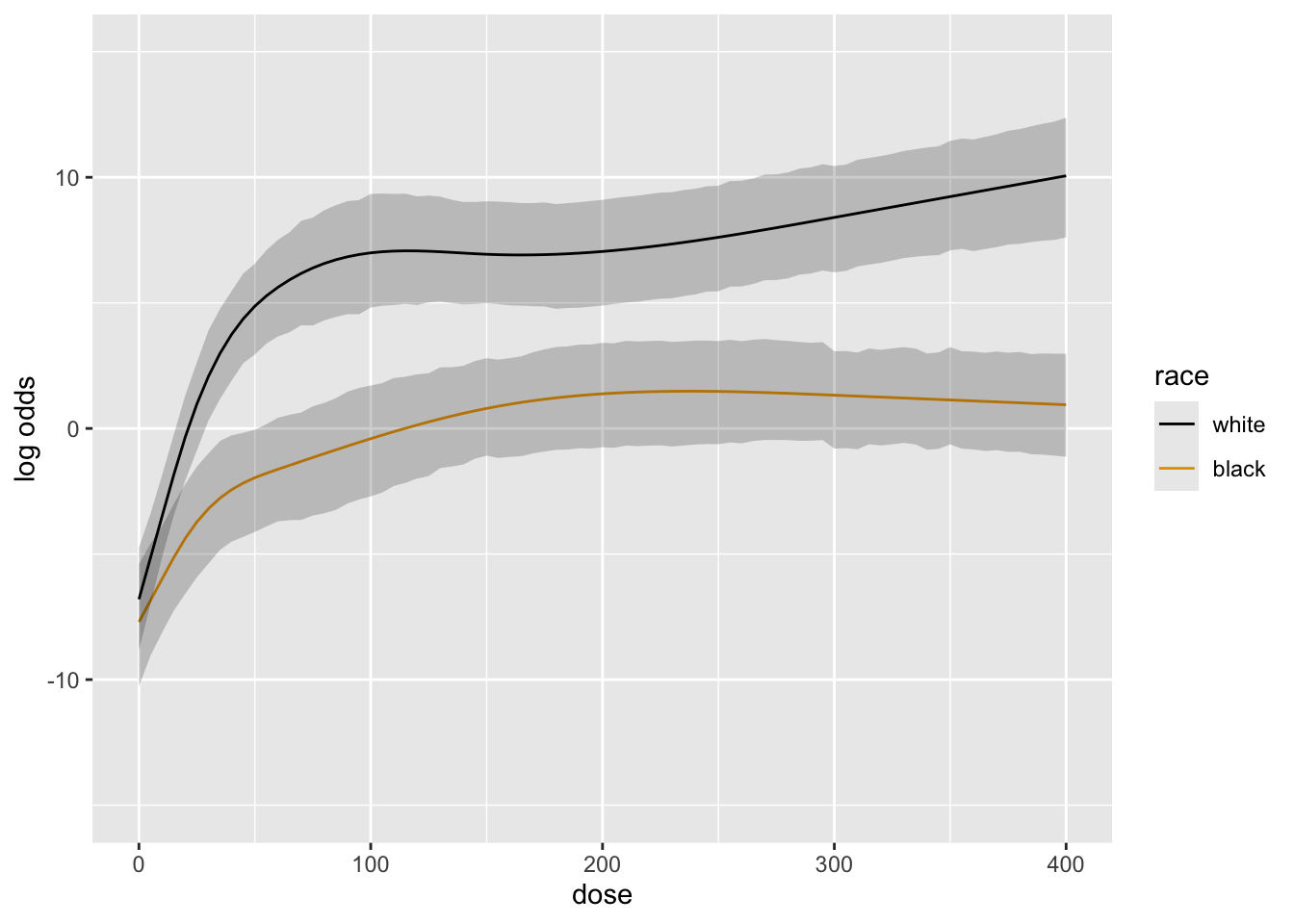Figure 15.9: Posterior mean logit of exceedance probability
Code
M <- Mean(b)
ggplot(Predict(b, dose=doses, race, fun=M))
k <- contrast(b, list(race='white', dose=seq(0, 400, by=100)),
list(race='black', dose=seq(0, 400, by=100)))
k
  dose  Contrast     S.E.     Lower     Upper Pr(Contrast>0)
1    0 0.9334039 1.346797 -1.543606  3.724625         0.7620
2  100 7.3840547 1.620470  4.227260 10.534312         1.0000
3  200 5.6608737 1.469336  2.877313  8.555347         0.9998
4  300 7.0756117 1.383830  4.419458  9.727653         1.0000
5  400 9.1159457 1.540959  6.226875 12.155031         1.0000

Intervals are 0.95 highest posterior density intervals
Contrast is the posterior mean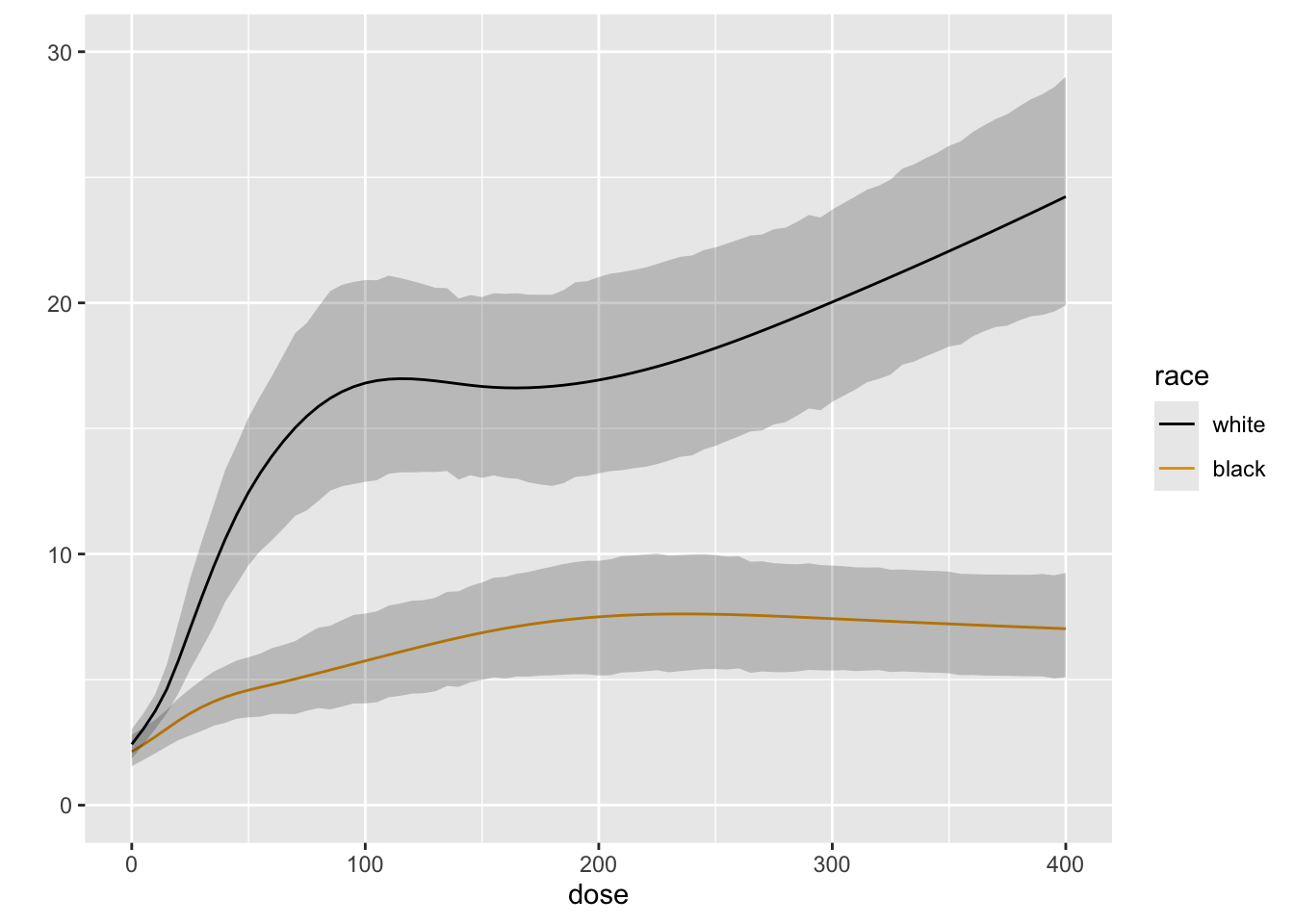Figure 15.10: Posterior mean of true mean forearm blood flow
Diggle, P. J., Heagerty, P., Liang, K.-Y., & Zeger, S. L. (2002). Analysis of Longitudinal Data (second). Oxford University Press.
Dupont, W. D. (2008). Statistical Modeling for Biomedical Researchers (second). Cambridge University Press.
Matthews, J. N. S., Altman, D. G., Campbell, M. J., & Royston, P. (1990). Analysis of serial measurements in medical research. BMJ, 300, 230–235. https://doi.org/10.1136/bmj.300.6719.230
O’Brien, P. C. (1988). Comparing two samples: Extensions of the t, rank-sum, and log-rank test. J Am Stat Assoc, 83, 52–61. https://doi.org/10.1080/01621459.1988.10478564
see Hauck WW, Hyslop T, Anderson S (2000) Stat in Med 19:887-899
Senn, S., Stevens, L., & Chaturvedi, N. (2000). Repeated measures in clinical trials: Simple strategies for analysis using summary measures. Stat Med, 19, 861–877. https://doi.org/10.1002/(SICI)1097-0258(20000330)19:6<861::AID-SIM407>3.0.CO;2-F